Скачать презентацию CHAPTER 8 Aggregate Expenditure and Equilibrium Output Prepared

d231c0c10ac31c444292c2e9ff2eaf04.ppt

• Количество слайдов: 41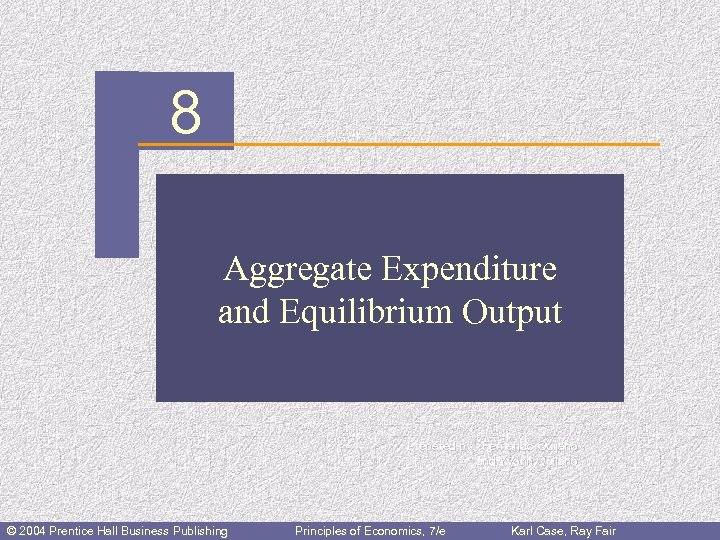CHAPTER 8 Aggregate Expenditure and Equilibrium Output Prepared by: Fernando Quijano and Yvonn Quijano © 2004 Prentice Hall Business Publishing Principles of Economics, 7/e Karl Case, Ray Fair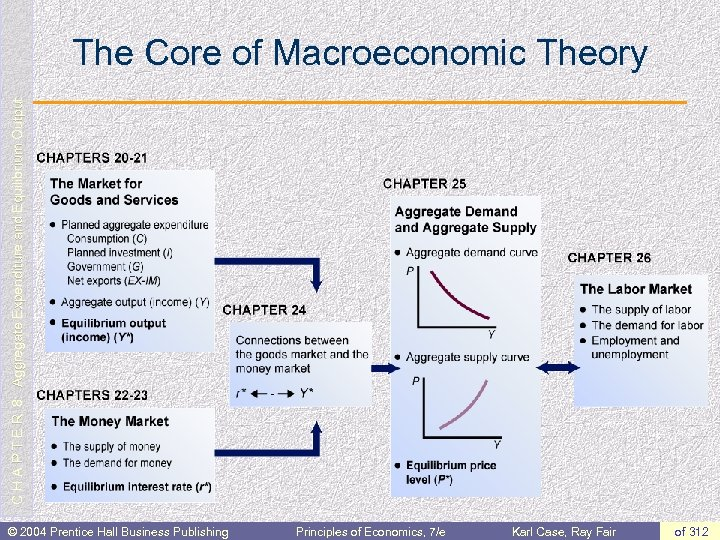C H A P T E R 8: Aggregate Expenditure and Equilibrium Output The Core of Macroeconomic Theory © 2004 Prentice Hall Business Publishing Principles of Economics, 7/e Karl Case, Ray Fair of 312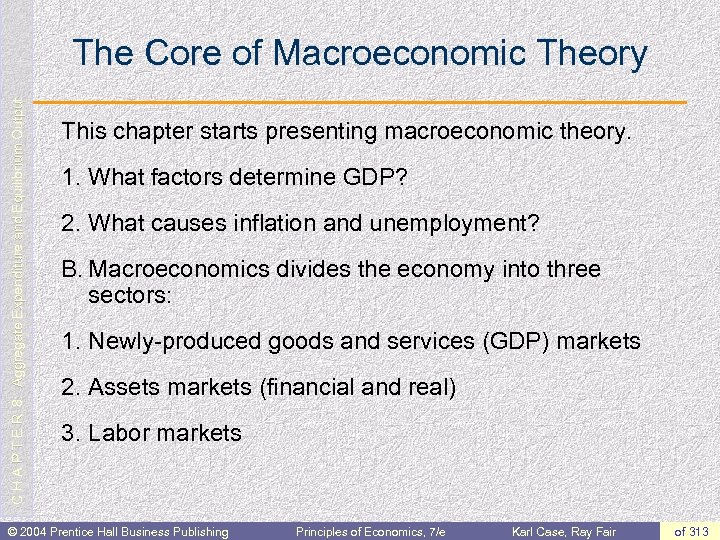C H A P T E R 8: Aggregate Expenditure and Equilibrium Output The Core of Macroeconomic Theory This chapter starts presenting macroeconomic theory. 1. What factors determine GDP? 2. What causes inflation and unemployment? B. Macroeconomics divides the economy into three sectors: 1. Newly-produced goods and services (GDP) markets 2. Assets markets (financial and real) 3. Labor markets © 2004 Prentice Hall Business Publishing Principles of Economics, 7/e Karl Case, Ray Fair of 313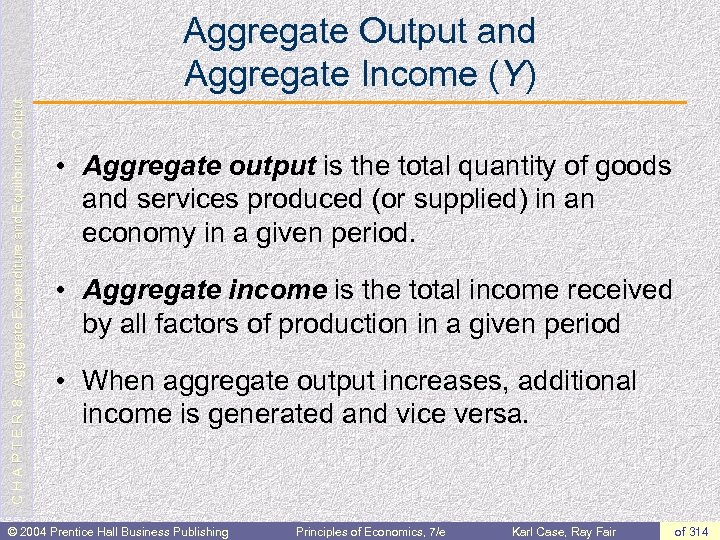C H A P T E R 8: Aggregate Expenditure and Equilibrium Output Aggregate Output and Aggregate Income (Y) • Aggregate output is the total quantity of goods and services produced (or supplied) in an economy in a given period. • Aggregate income is the total income received by all factors of production in a given period • When aggregate output increases, additional income is generated and vice versa. © 2004 Prentice Hall Business Publishing Principles of Economics, 7/e Karl Case, Ray Fair of 314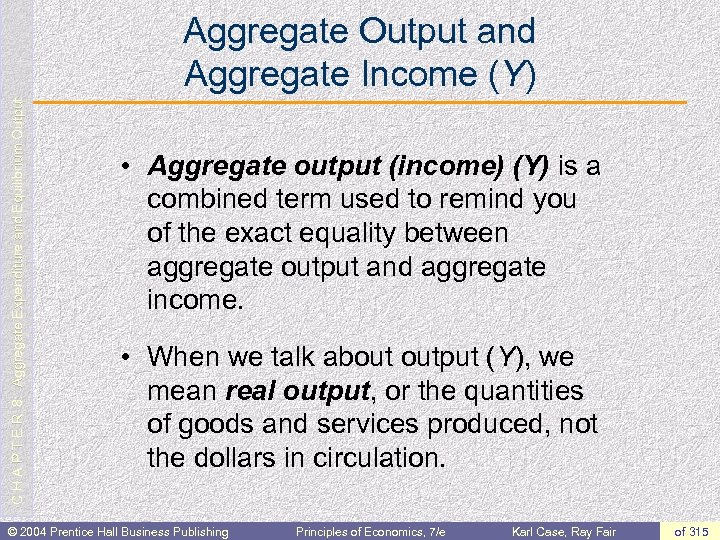C H A P T E R 8: Aggregate Expenditure and Equilibrium Output Aggregate Output and Aggregate Income (Y) • Aggregate output (income) (Y) is a combined term used to remind you of the exact equality between aggregate output and aggregate income. • When we talk about output (Y), we mean real output, or the quantities of goods and services produced, not the dollars in circulation. © 2004 Prentice Hall Business Publishing Principles of Economics, 7/e Karl Case, Ray Fair of 315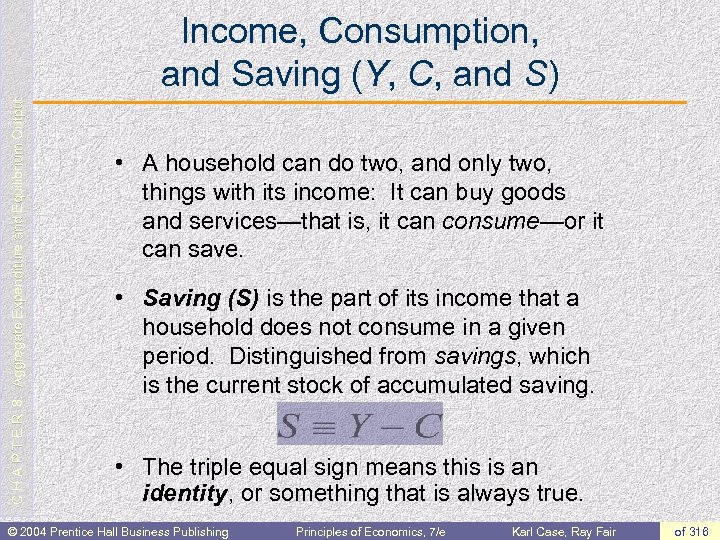C H A P T E R 8: Aggregate Expenditure and Equilibrium Output Income, Consumption, and Saving (Y, C, and S) • A household can do two, and only two, things with its income: It can buy goods and services—that is, it can consume—or it can save. • Saving (S) is the part of its income that a household does not consume in a given period. Distinguished from savings, which is the current stock of accumulated saving. • The triple equal sign means this is an identity, or something that is always true. © 2004 Prentice Hall Business Publishing Principles of Economics, 7/e Karl Case, Ray Fair of 316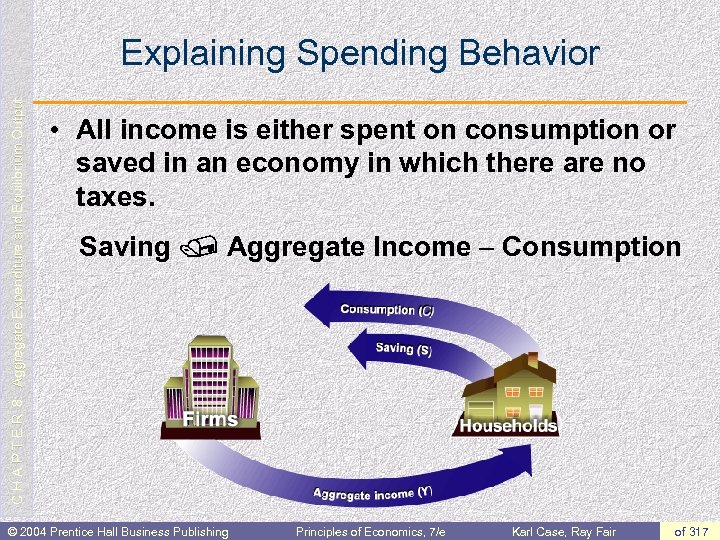C H A P T E R 8: Aggregate Expenditure and Equilibrium Output Explaining Spending Behavior • All income is either spent on consumption or saved in an economy in which there are no taxes. Saving / Aggregate Income - Consumption © 2004 Prentice Hall Business Publishing Principles of Economics, 7/e Karl Case, Ray Fair of 317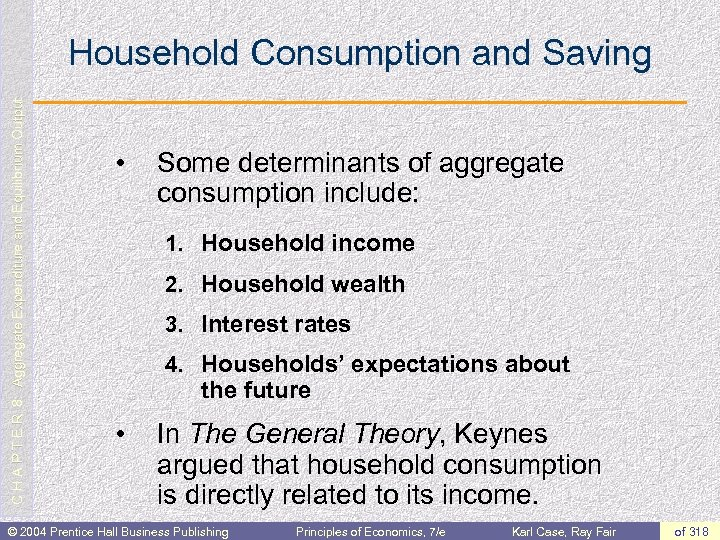C H A P T E R 8: Aggregate Expenditure and Equilibrium Output Household Consumption and Saving • Some determinants of aggregate consumption include: 1. Household income 2. Household wealth 3. Interest rates 4. Households’ expectations about the future • In The General Theory, Keynes argued that household consumption is directly related to its income. © 2004 Prentice Hall Business Publishing Principles of Economics, 7/e Karl Case, Ray Fair of 318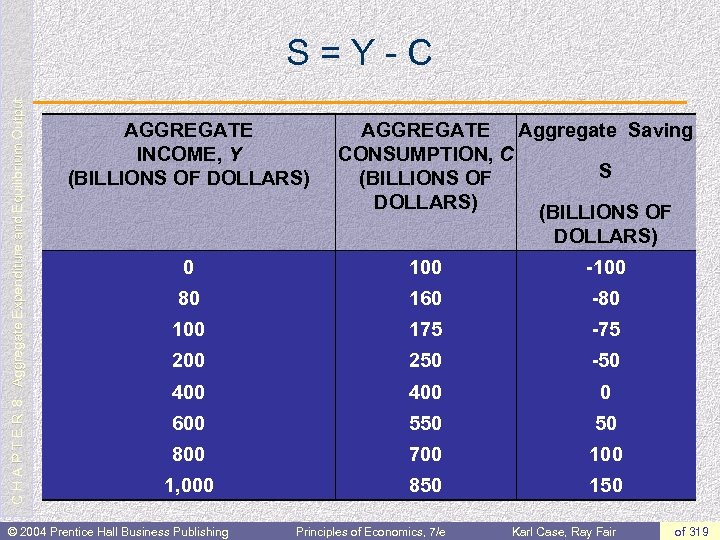C H A P T E R 8: Aggregate Expenditure and Equilibrium Output S=Y-C AGGREGATE INCOME, Y (BILLIONS OF DOLLARS) AGGREGATE Aggregate Saving CONSUMPTION, C S (BILLIONS OF DOLLARS) 0 100 -100 80 160 -80 100 175 -75 200 250 -50 400 0 600 550 50 800 700 1, 000 850 150 © 2004 Prentice Hall Business Publishing Principles of Economics, 7/e Karl Case, Ray Fair of 319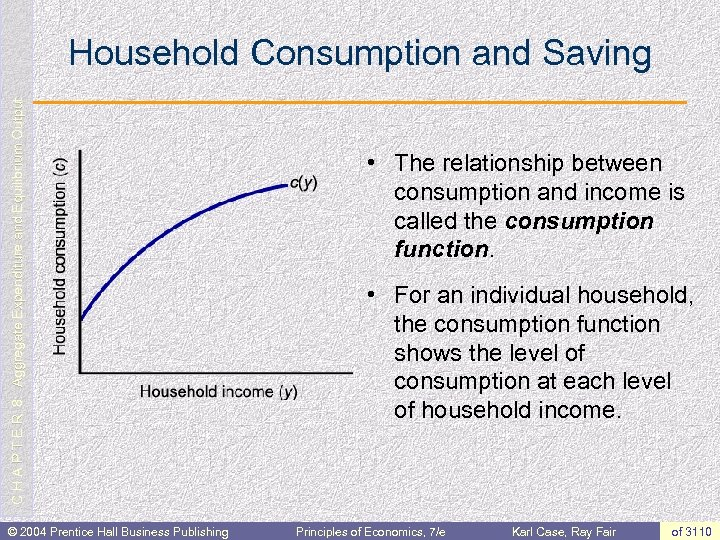C H A P T E R 8: Aggregate Expenditure and Equilibrium Output Household Consumption and Saving © 2004 Prentice Hall Business Publishing • The relationship between consumption and income is called the consumption function. • For an individual household, the consumption function shows the level of consumption at each level of household income. Principles of Economics, 7/e Karl Case, Ray Fair of 3110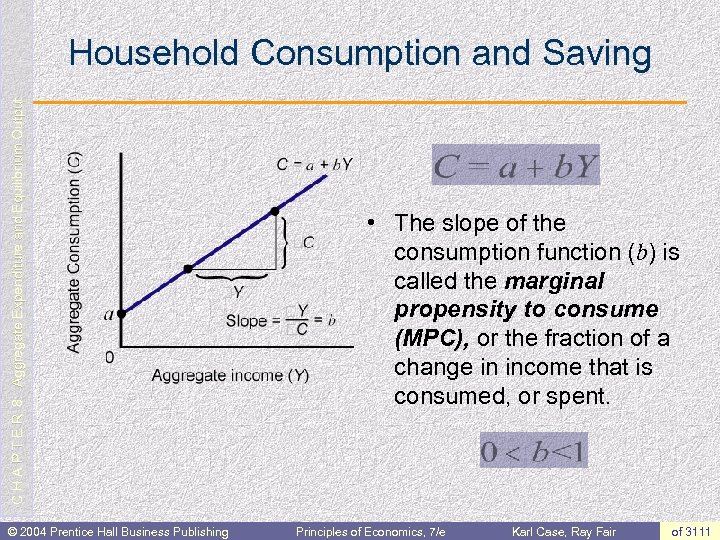C H A P T E R 8: Aggregate Expenditure and Equilibrium Output Household Consumption and Saving © 2004 Prentice Hall Business Publishing • The slope of the consumption function (b) is called the marginal propensity to consume (MPC), or the fraction of a change in income that is consumed, or spent. Principles of Economics, 7/e Karl Case, Ray Fair of 3111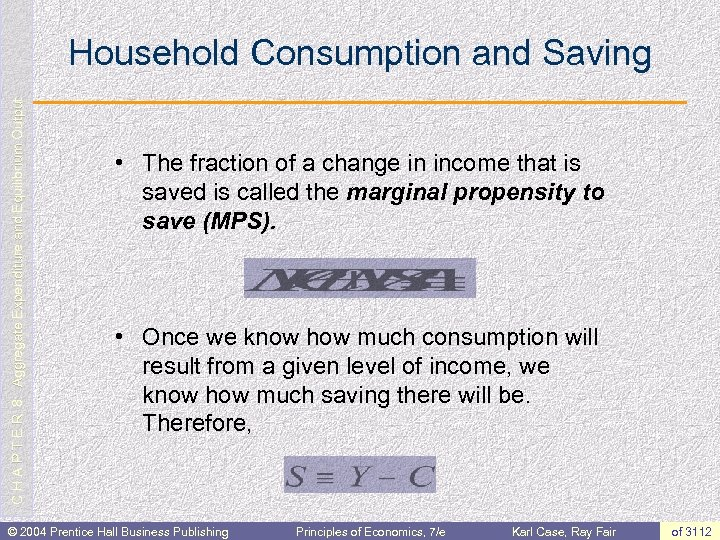C H A P T E R 8: Aggregate Expenditure and Equilibrium Output Household Consumption and Saving • The fraction of a change in income that is saved is called the marginal propensity to save (MPS). • Once we know how much consumption will result from a given level of income, we know how much saving there will be. Therefore, © 2004 Prentice Hall Business Publishing Principles of Economics, 7/e Karl Case, Ray Fair of 3112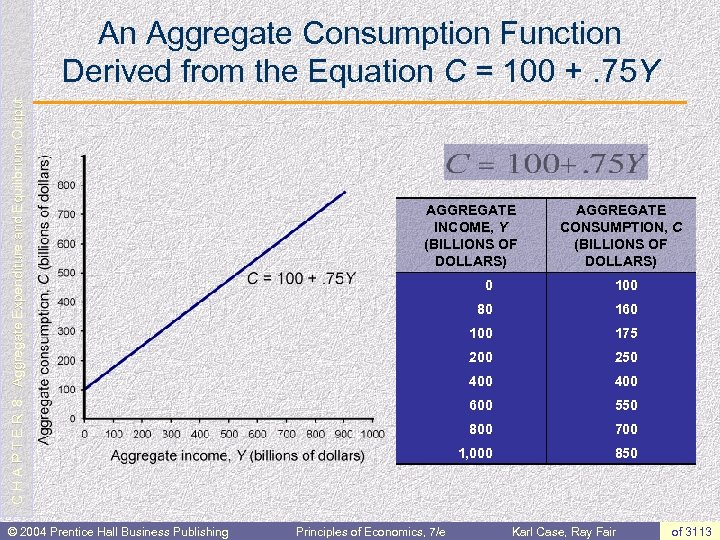C H A P T E R 8: Aggregate Expenditure and Equilibrium Output An Aggregate Consumption Function Derived from the Equation C = 100 +. 75 Y © 2004 Prentice Hall Business Publishing AGGREGATE INCOME, Y (BILLIONS OF DOLLARS) AGGREGATE CONSUMPTION, C (BILLIONS OF DOLLARS) 0 80 160 100 175 200 250 400 600 550 800 700 1, 000 Principles of Economics, 7/e 100 850 Karl Case, Ray Fair of 3113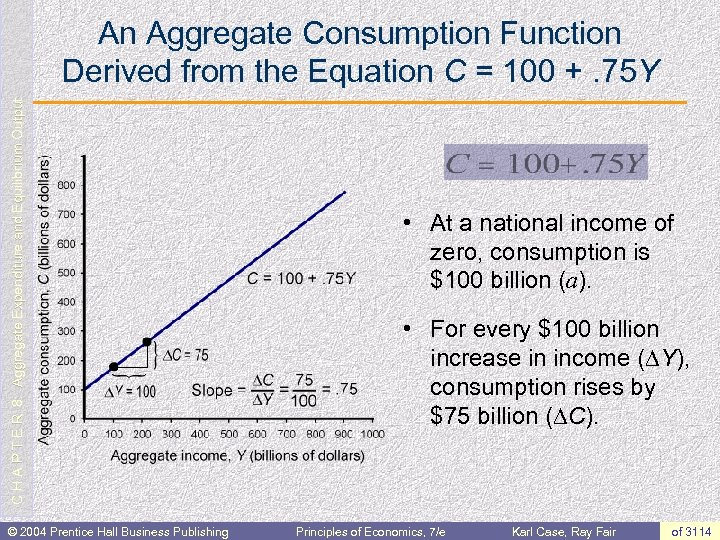C H A P T E R 8: Aggregate Expenditure and Equilibrium Output An Aggregate Consumption Function Derived from the Equation C = 100 +. 75 Y © 2004 Prentice Hall Business Publishing • At a national income of zero, consumption is \$100 billion (a). • For every \$100 billion increase in income (DY), consumption rises by \$75 billion (DC). Principles of Economics, 7/e Karl Case, Ray Fair of 3114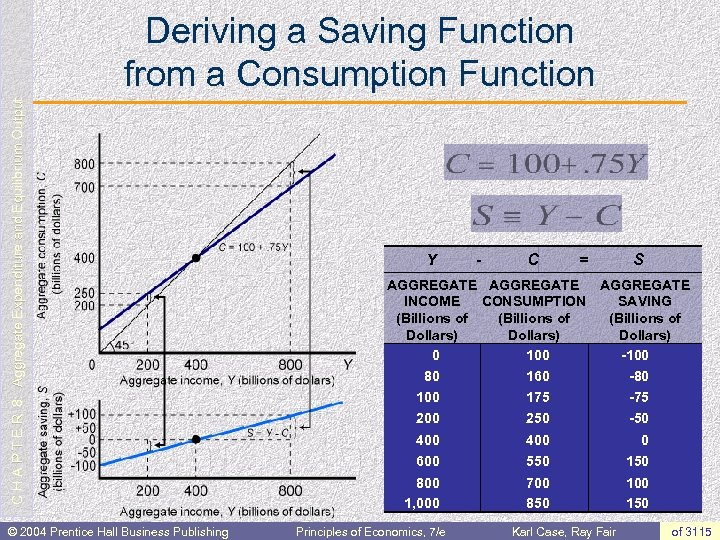C H A P T E R 8: Aggregate Expenditure and Equilibrium Output Deriving a Saving Function from a Consumption Function © 2004 Prentice Hall Business Publishing Y - C = S AGGREGATE INCOME CONSUMPTION SAVING (Billions of Dollars) 0 100 -100 80 160 -80 100 175 -75 200 250 -50 400 0 600 550 150 800 1, 000 700 850 100 150 Principles of Economics, 7/e Karl Case, Ray Fair of 3115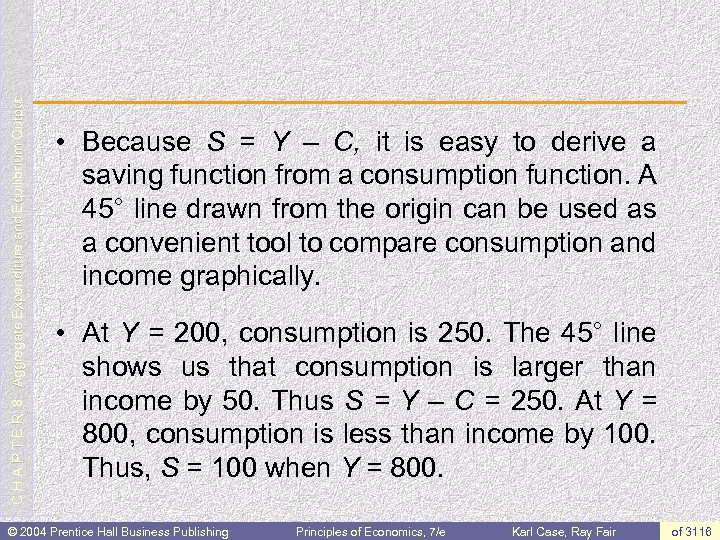C H A P T E R 8: Aggregate Expenditure and Equilibrium Output • Because S = Y – C, it is easy to derive a saving function from a consumption function. A 45° line drawn from the origin can be used as a convenient tool to compare consumption and income graphically. • At Y = 200, consumption is 250. The 45° line shows us that consumption is larger than income by 50. Thus S = Y – C = 250. At Y = 800, consumption is less than income by 100. Thus, S = 100 when Y = 800. © 2004 Prentice Hall Business Publishing Principles of Economics, 7/e Karl Case, Ray Fair of 3116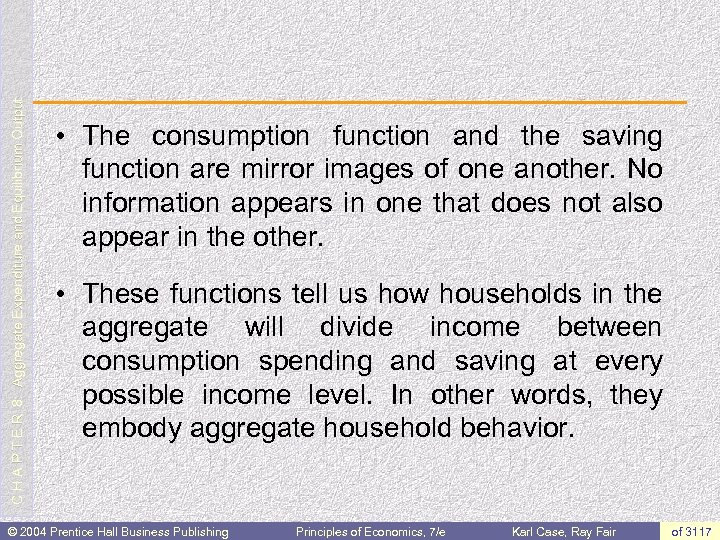C H A P T E R 8: Aggregate Expenditure and Equilibrium Output • The consumption function and the saving function are mirror images of one another. No information appears in one that does not also appear in the other. • These functions tell us how households in the aggregate will divide income between consumption spending and saving at every possible income level. In other words, they embody aggregate household behavior. © 2004 Prentice Hall Business Publishing Principles of Economics, 7/e Karl Case, Ray Fair of 3117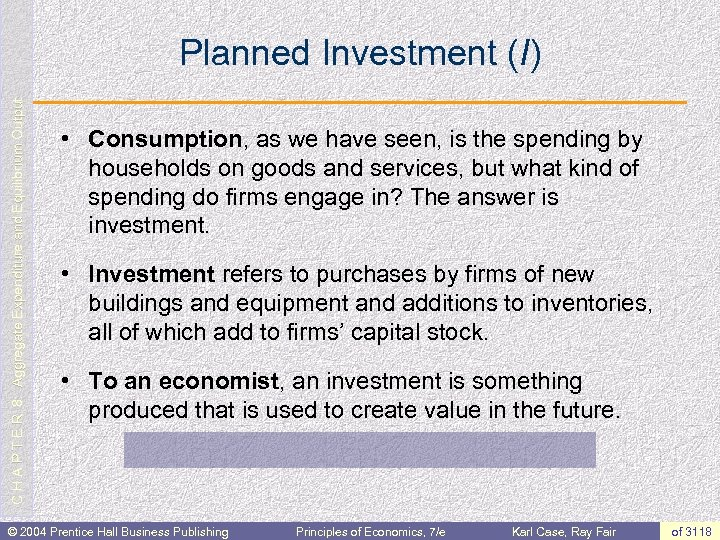C H A P T E R 8: Aggregate Expenditure and Equilibrium Output Planned Investment (I) • Consumption, as we have seen, is the spending by households on goods and services, but what kind of spending do firms engage in? The answer is investment. • Investment refers to purchases by firms of new buildings and equipment and additions to inventories, all of which add to firms’ capital stock. • To an economist, an investment is something produced that is used to create value in the future. © 2004 Prentice Hall Business Publishing Principles of Economics, 7/e Karl Case, Ray Fair of 3118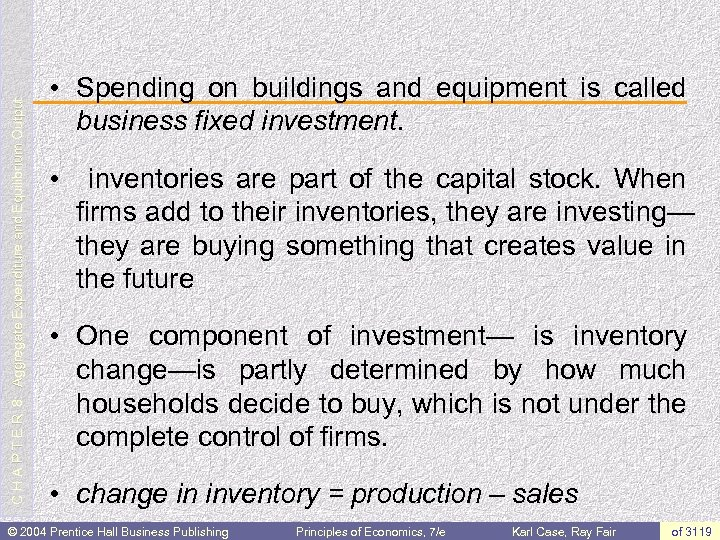C H A P T E R 8: Aggregate Expenditure and Equilibrium Output • Spending on buildings and equipment is called business fixed investment. • inventories are part of the capital stock. When firms add to their inventories, they are investing— they are buying something that creates value in the future • One component of investment— is inventory change—is partly determined by how much households decide to buy, which is not under the complete control of firms. • change in inventory = production – sales © 2004 Prentice Hall Business Publishing Principles of Economics, 7/e Karl Case, Ray Fair of 3119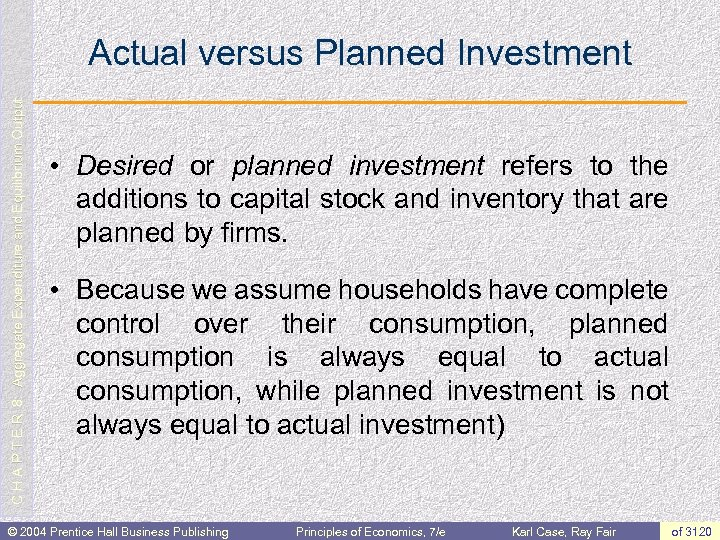C H A P T E R 8: Aggregate Expenditure and Equilibrium Output Actual versus Planned Investment • Desired or planned investment refers to the additions to capital stock and inventory that are planned by firms. • Because we assume households have complete control over their consumption, planned consumption is always equal to actual consumption, while planned investment is not always equal to actual investment) © 2004 Prentice Hall Business Publishing Principles of Economics, 7/e Karl Case, Ray Fair of 3120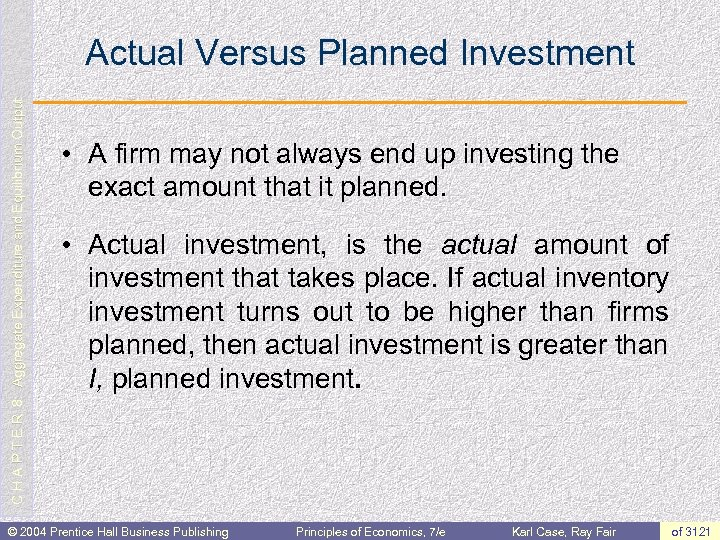C H A P T E R 8: Aggregate Expenditure and Equilibrium Output Actual Versus Planned Investment • A firm may not always end up investing the exact amount that it planned. • Actual investment, is the actual amount of investment that takes place. If actual inventory investment turns out to be higher than firms planned, then actual investment is greater than I, planned investment. © 2004 Prentice Hall Business Publishing Principles of Economics, 7/e Karl Case, Ray Fair of 3121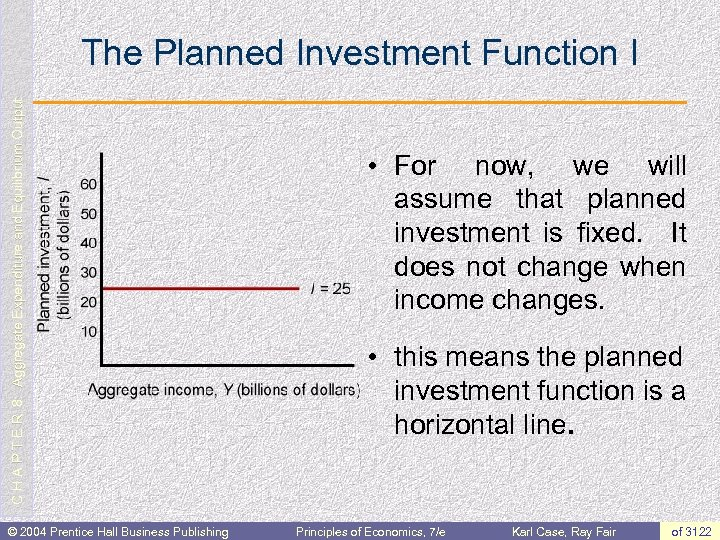C H A P T E R 8: Aggregate Expenditure and Equilibrium Output The Planned Investment Function I © 2004 Prentice Hall Business Publishing • For now, we will assume that planned investment is fixed. It does not change when income changes. • this means the planned investment function is a horizontal line. Principles of Economics, 7/e Karl Case, Ray Fair of 3122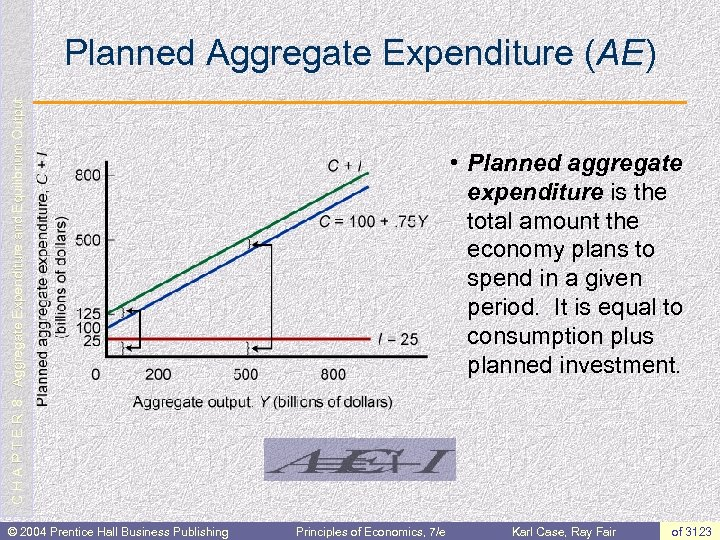C H A P T E R 8: Aggregate Expenditure and Equilibrium Output Planned Aggregate Expenditure (AE) © 2004 Prentice Hall Business Publishing • Planned aggregate expenditure is the total amount the economy plans to spend in a given period. It is equal to consumption plus planned investment. Principles of Economics, 7/e Karl Case, Ray Fair of 3123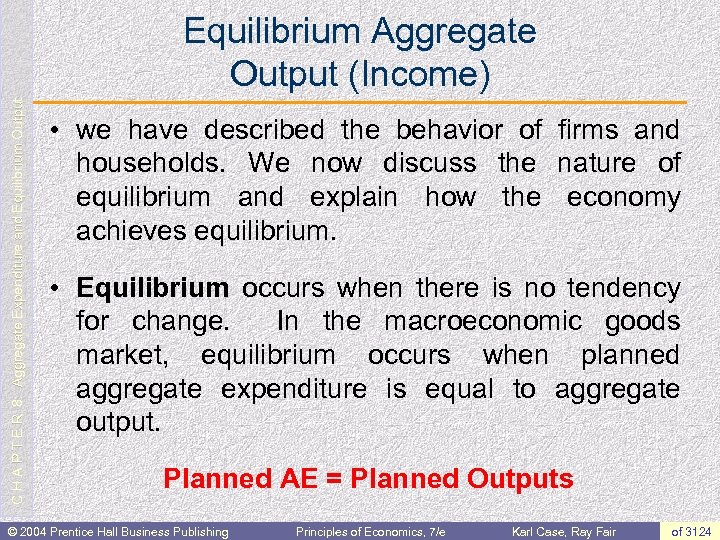C H A P T E R 8: Aggregate Expenditure and Equilibrium Output Equilibrium Aggregate Output (Income) • we have described the behavior of firms and households. We now discuss the nature of equilibrium and explain how the economy achieves equilibrium. • Equilibrium occurs when there is no tendency for change. In the macroeconomic goods market, equilibrium occurs when planned aggregate expenditure is equal to aggregate output. Planned AE = Planned Outputs © 2004 Prentice Hall Business Publishing Principles of Economics, 7/e Karl Case, Ray Fair of 3124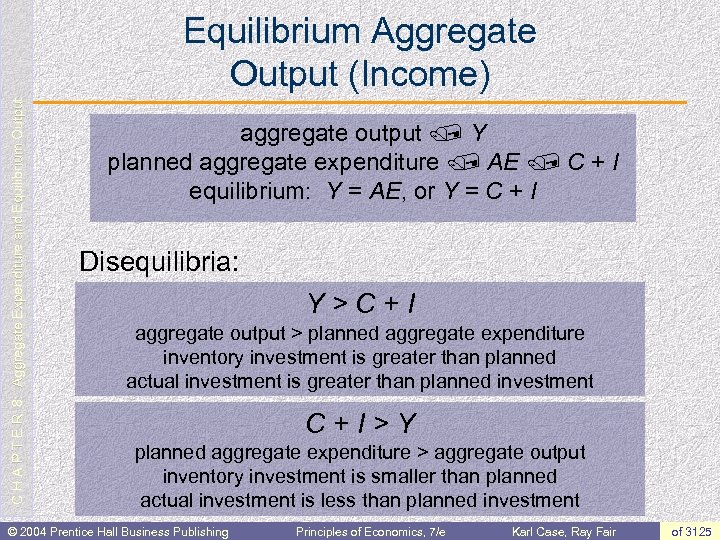C H A P T E R 8: Aggregate Expenditure and Equilibrium Output Equilibrium Aggregate Output (Income) aggregate output / Y planned aggregate expenditure / AE / C + I equilibrium: Y = AE, or Y = C + I Disequilibria: Y>C+I aggregate output > planned aggregate expenditure inventory investment is greater than planned actual investment is greater than planned investment C+I>Y planned aggregate expenditure > aggregate output inventory investment is smaller than planned actual investment is less than planned investment © 2004 Prentice Hall Business Publishing Principles of Economics, 7/e Karl Case, Ray Fair of 3125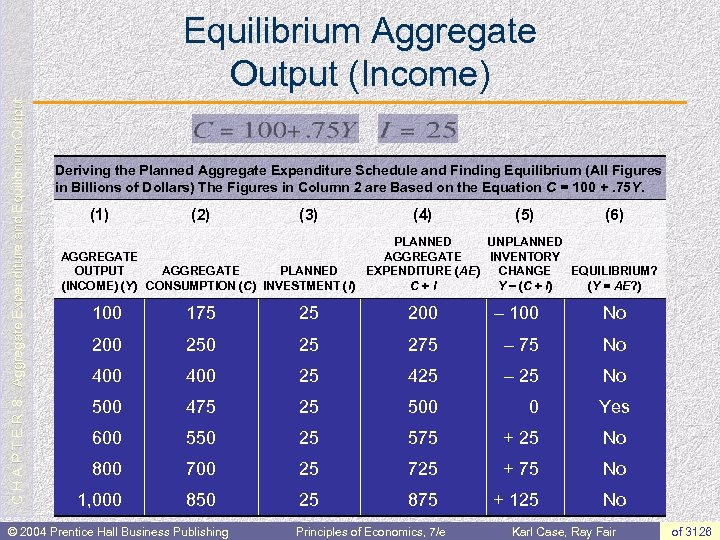C H A P T E R 8: Aggregate Expenditure and Equilibrium Output Equilibrium Aggregate Output (Income) Deriving the Planned Aggregate Expenditure Schedule and Finding Equilibrium (All Figures in Billions of Dollars) The Figures in Column 2 are Based on the Equation C = 100 +. 75 Y. (1) (2) (3) (4) (5) (6) PLANNED UNPLANNED AGGREGATE INVENTORY OUTPUT AGGREGATE PLANNED EXPENDITURE (AE) CHANGE EQUILIBRIUM? (INCOME) (Y) CONSUMPTION (C) INVESTMENT (I) C+I Y - (C + I) (Y = AE? ) 100 175 25 200 - 100 No 200 25 275 - 75 No 400 25 425 - 25 No 500 475 25 500 0 Yes 600 550 25 575 + 25 No 800 700 25 725 + 75 No 1, 000 850 25 875 + 125 No © 2004 Prentice Hall Business Publishing Principles of Economics, 7/e Karl Case, Ray Fair of 3126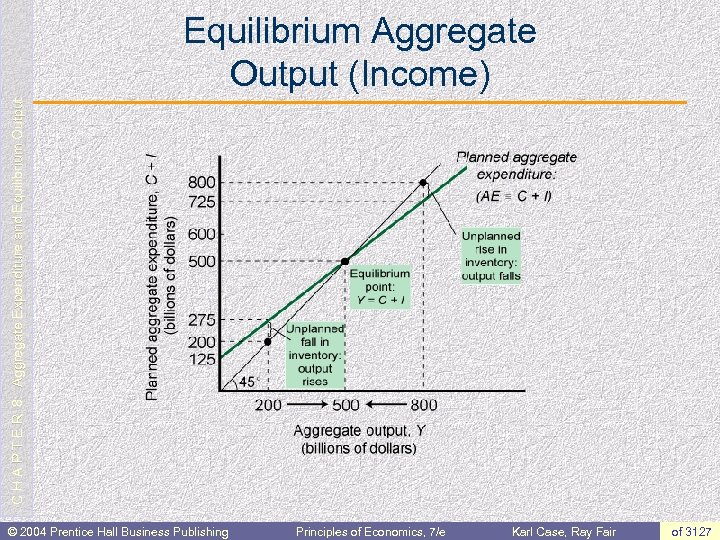C H A P T E R 8: Aggregate Expenditure and Equilibrium Output Equilibrium Aggregate Output (Income) © 2004 Prentice Hall Business Publishing Principles of Economics, 7/e Karl Case, Ray Fair of 3127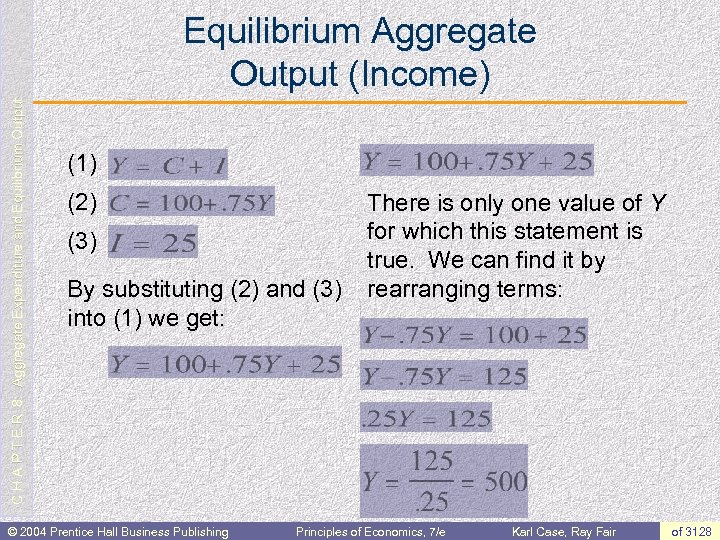C H A P T E R 8: Aggregate Expenditure and Equilibrium Output Equilibrium Aggregate Output (Income) (1) (2) (3) By substituting (2) and (3) into (1) we get: © 2004 Prentice Hall Business Publishing There is only one value of Y for which this statement is true. We can find it by rearranging terms: Principles of Economics, 7/e Karl Case, Ray Fair of 3128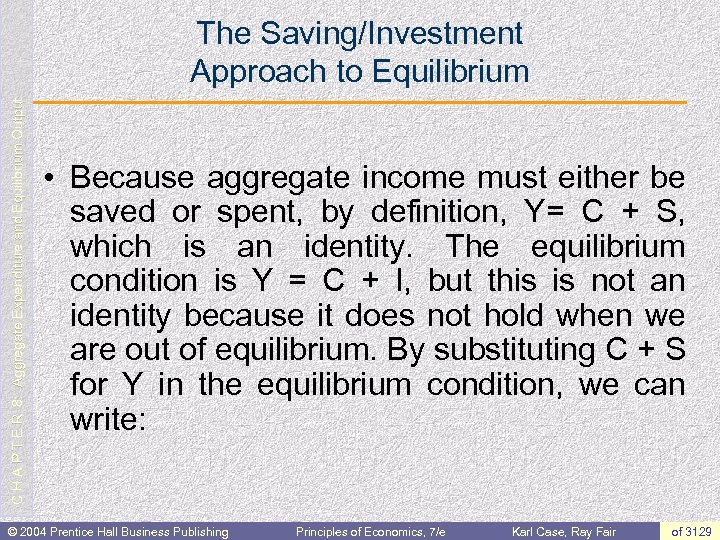C H A P T E R 8: Aggregate Expenditure and Equilibrium Output The Saving/Investment Approach to Equilibrium • Because aggregate income must either be saved or spent, by definition, Y= C + S, which is an identity. The equilibrium condition is Y = C + I, but this is not an identity because it does not hold when we are out of equilibrium. By substituting C + S for Y in the equilibrium condition, we can write: © 2004 Prentice Hall Business Publishing Principles of Economics, 7/e Karl Case, Ray Fair of 3129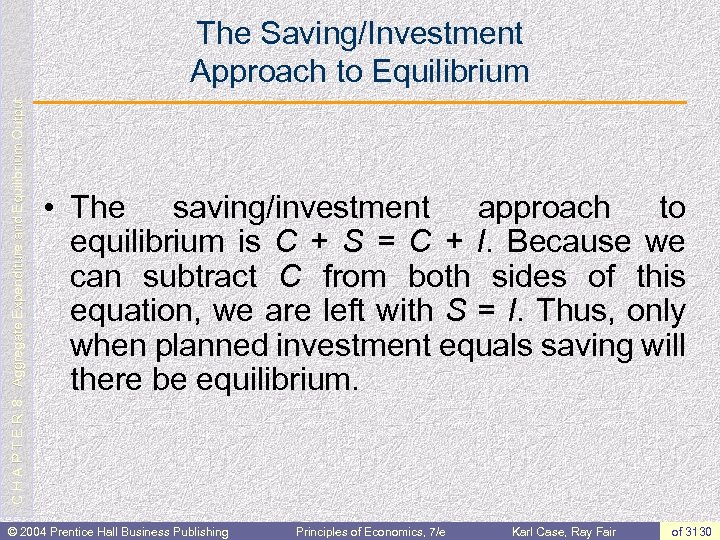C H A P T E R 8: Aggregate Expenditure and Equilibrium Output The Saving/Investment Approach to Equilibrium • The saving/investment approach to equilibrium is C + S = C + I. Because we can subtract C from both sides of this equation, we are left with S = I. Thus, only when planned investment equals saving will there be equilibrium. © 2004 Prentice Hall Business Publishing Principles of Economics, 7/e Karl Case, Ray Fair of 3130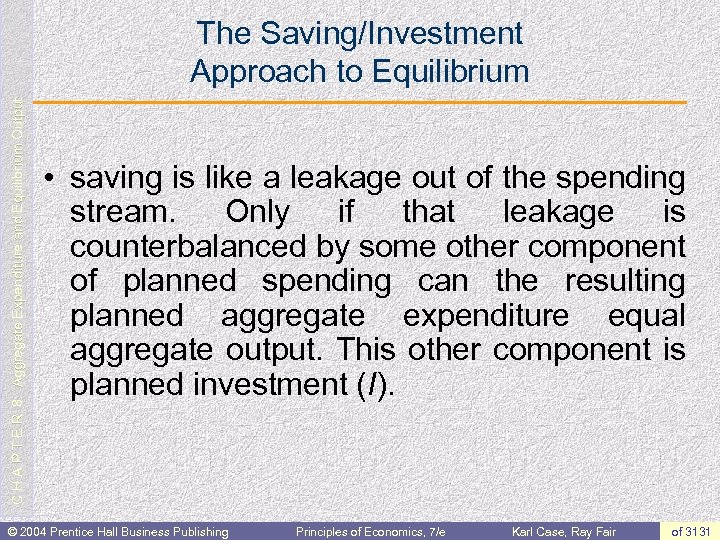C H A P T E R 8: Aggregate Expenditure and Equilibrium Output The Saving/Investment Approach to Equilibrium • saving is like a leakage out of the spending stream. Only if that leakage is counterbalanced by some other component of planned spending can the resulting planned aggregate expenditure equal aggregate output. This other component is planned investment (I). © 2004 Prentice Hall Business Publishing Principles of Economics, 7/e Karl Case, Ray Fair of 3131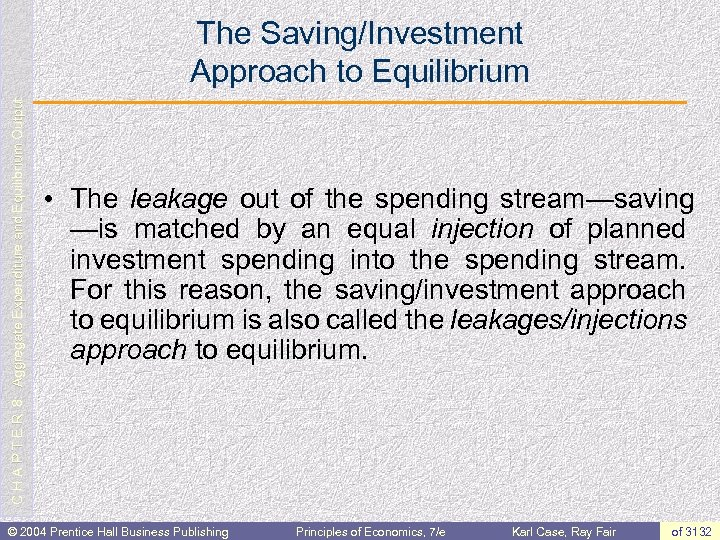C H A P T E R 8: Aggregate Expenditure and Equilibrium Output The Saving/Investment Approach to Equilibrium • The leakage out of the spending stream—saving —is matched by an equal injection of planned investment spending into the spending stream. For this reason, the saving/investment approach to equilibrium is also called the leakages/injections approach to equilibrium. © 2004 Prentice Hall Business Publishing Principles of Economics, 7/e Karl Case, Ray Fair of 3132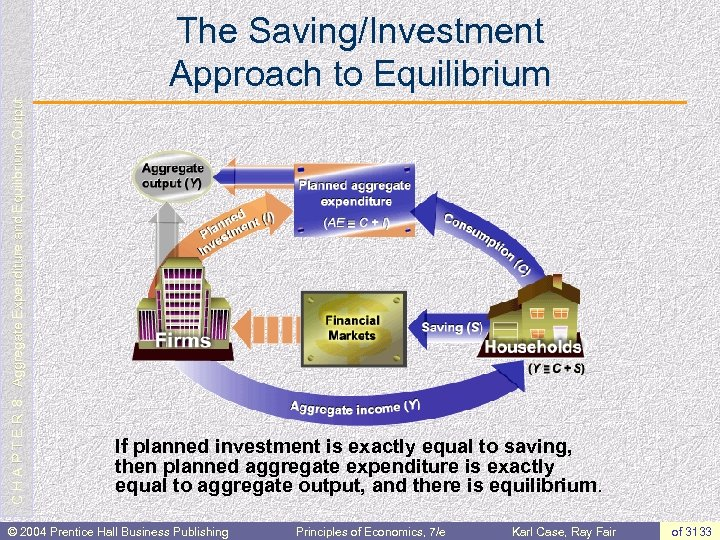C H A P T E R 8: Aggregate Expenditure and Equilibrium Output The Saving/Investment Approach to Equilibrium If planned investment is exactly equal to saving, then planned aggregate expenditure is exactly equal to aggregate output, and there is equilibrium. © 2004 Prentice Hall Business Publishing Principles of Economics, 7/e Karl Case, Ray Fair of 3133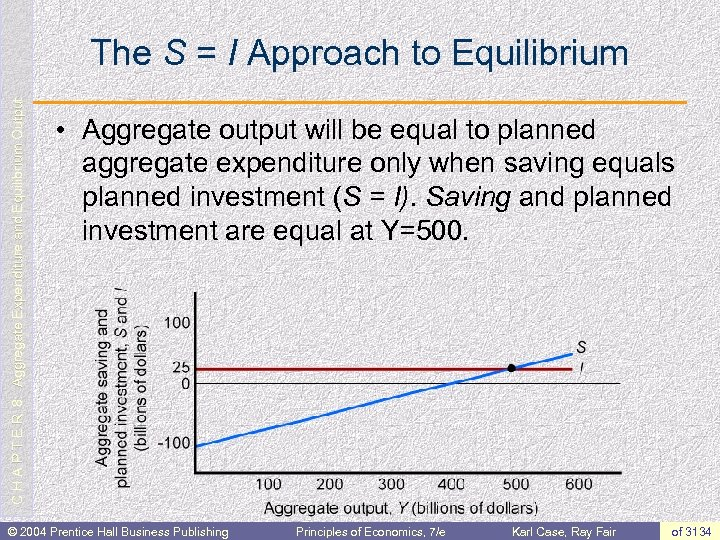C H A P T E R 8: Aggregate Expenditure and Equilibrium Output The S = I Approach to Equilibrium • Aggregate output will be equal to planned aggregate expenditure only when saving equals planned investment (S = I). Saving and planned investment are equal at Y=500. © 2004 Prentice Hall Business Publishing Principles of Economics, 7/e Karl Case, Ray Fair of 3134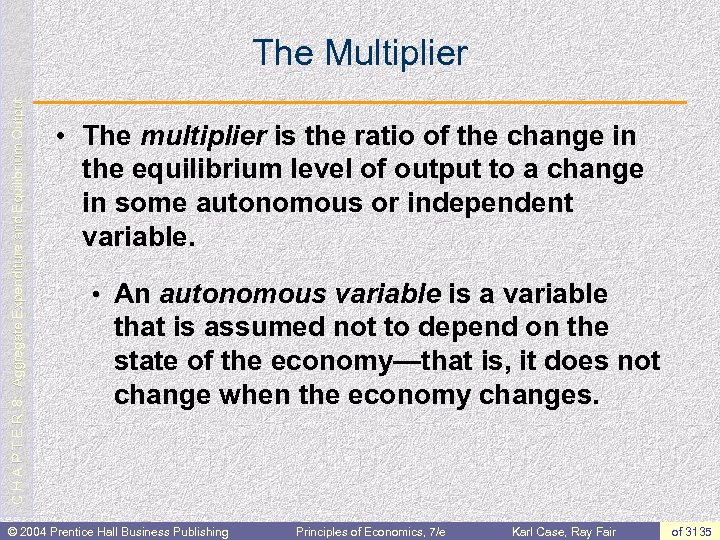C H A P T E R 8: Aggregate Expenditure and Equilibrium Output The Multiplier • The multiplier is the ratio of the change in the equilibrium level of output to a change in some autonomous or independent variable. • An autonomous variable is a variable that is assumed not to depend on the state of the economy—that is, it does not change when the economy changes. © 2004 Prentice Hall Business Publishing Principles of Economics, 7/e Karl Case, Ray Fair of 3135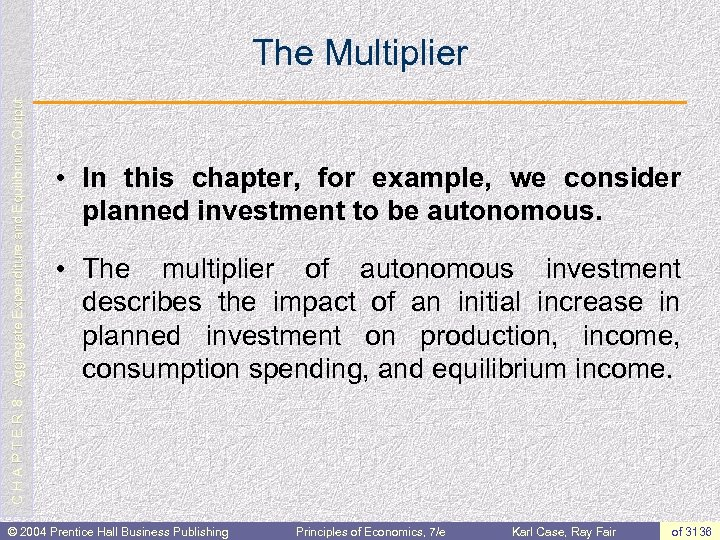C H A P T E R 8: Aggregate Expenditure and Equilibrium Output The Multiplier • In this chapter, for example, we consider planned investment to be autonomous. • The multiplier of autonomous investment describes the impact of an initial increase in planned investment on production, income, consumption spending, and equilibrium income. © 2004 Prentice Hall Business Publishing Principles of Economics, 7/e Karl Case, Ray Fair of 3136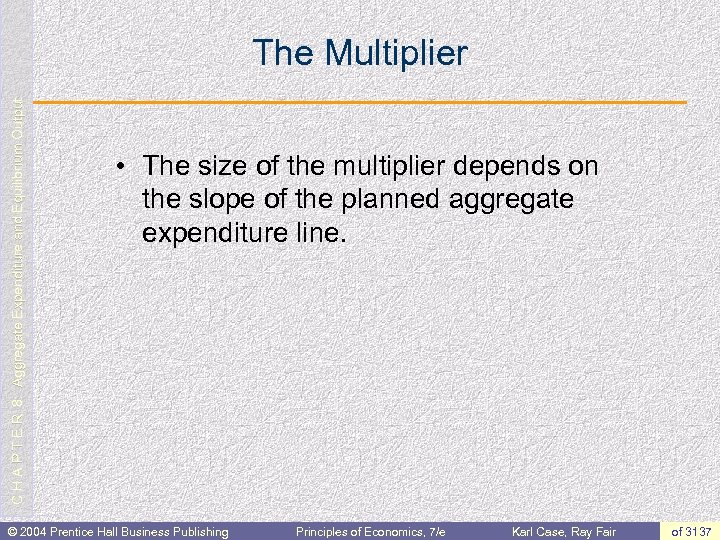C H A P T E R 8: Aggregate Expenditure and Equilibrium Output The Multiplier • The size of the multiplier depends on the slope of the planned aggregate expenditure line. © 2004 Prentice Hall Business Publishing Principles of Economics, 7/e Karl Case, Ray Fair of 3137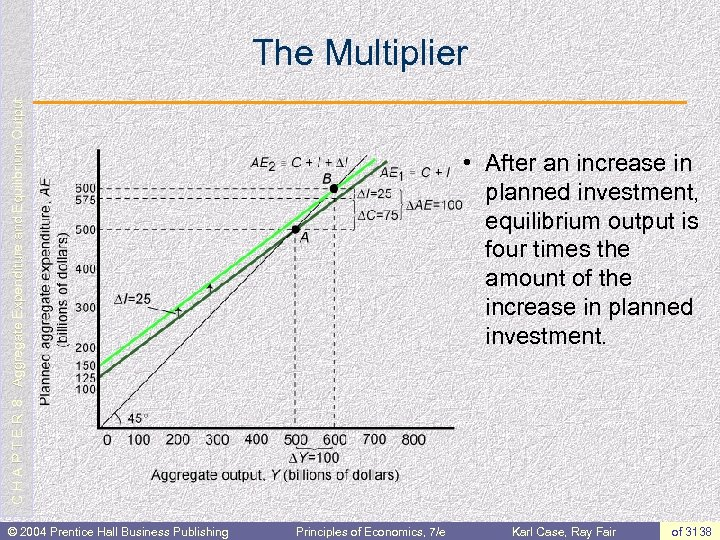C H A P T E R 8: Aggregate Expenditure and Equilibrium Output The Multiplier © 2004 Prentice Hall Business Publishing • After an increase in planned investment, equilibrium output is four times the amount of the increase in planned investment. Principles of Economics, 7/e Karl Case, Ray Fair of 3138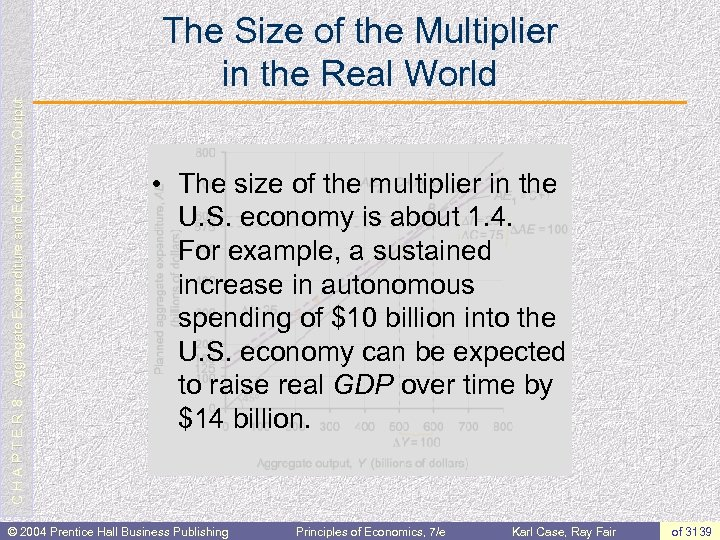C H A P T E R 8: Aggregate Expenditure and Equilibrium Output The Size of the Multiplier in the Real World • The size of the multiplier in the U. S. economy is about 1. 4. For example, a sustained increase in autonomous spending of \$10 billion into the U. S. economy can be expected to raise real GDP over time by \$14 billion. © 2004 Prentice Hall Business Publishing Principles of Economics, 7/e Karl Case, Ray Fair of 3139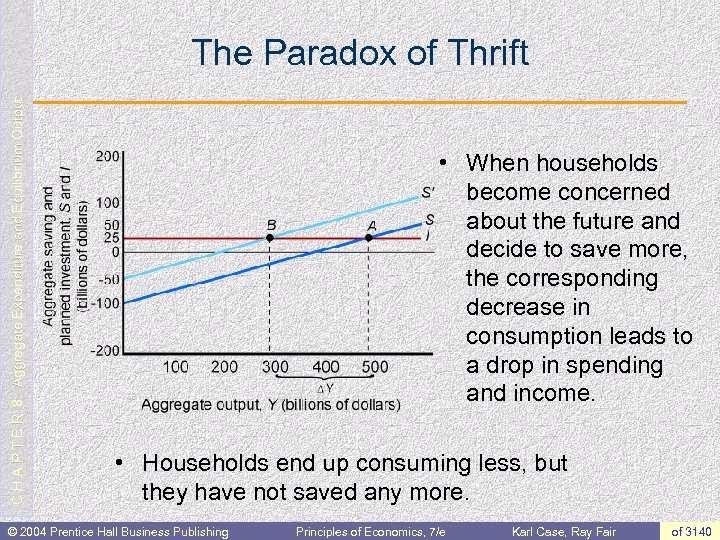C H A P T E R 8: Aggregate Expenditure and Equilibrium Output The Paradox of Thrift • When households become concerned about the future and decide to save more, the corresponding decrease in consumption leads to a drop in spending and income. • Households end up consuming less, but they have not saved any more. © 2004 Prentice Hall Business Publishing Principles of Economics, 7/e Karl Case, Ray Fair of 3140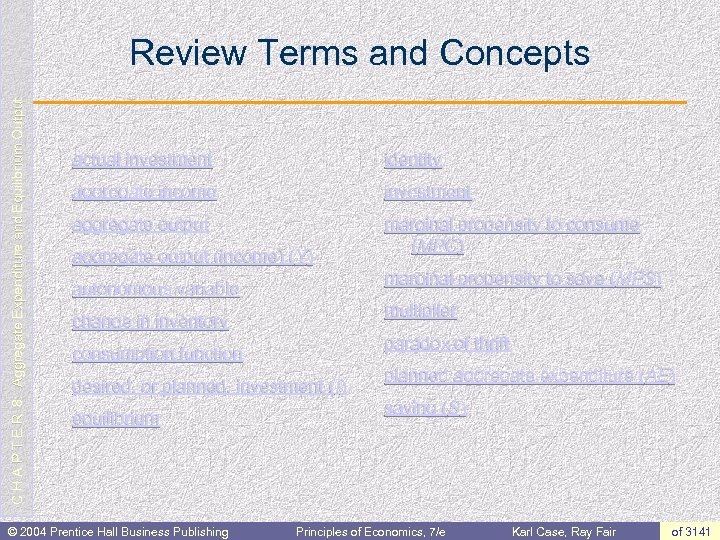C H A P T E R 8: Aggregate Expenditure and Equilibrium Output Review Terms and Concepts actual investment identity aggregate income investment aggregate output marginal propensity to consume (MPC) aggregate output (income) (Y) marginal propensity to save (MPS) autonomous variable multiplier change in inventory paradox of thrift consumption function desired, or planned, investment (I) equilibrium © 2004 Prentice Hall Business Publishing planned aggregate expenditure (AE) saving (S) Principles of Economics, 7/e Karl Case, Ray Fair of 3141